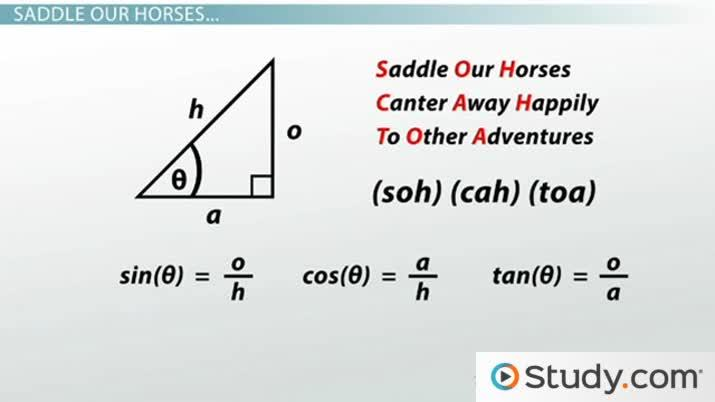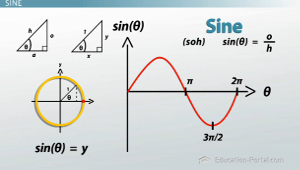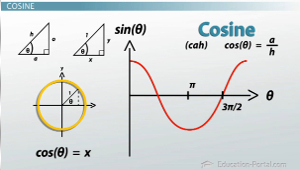Trigonometry: Sine and Cosine

Lesson Transcript
Instructor: Eric Garneau
Sine and cosine are basic trigonometric functions that are used to solve for the angles and sides of triangles. Review trigonometry concepts and learn about the mnemonic used for sine, cosine, and tangent functions. Updated: 10/23/2021

Trigonometry Review

Let's review some trigonometry. Let's say we have a unit circle - that is, a circle with a radius of 1 - and we're going to draw a point from the origin to the outside of the circle, some point x,y. The angle made between that line (between the origin and x,y) and the x-axis I'll call theta. What we're doing is basically taking a slice of pie out of our unit circle. Let's focus on that piece we just cut out. In particular, let's focus on the triangle made up of the radius 1, the x coordinate (which is one of the legs of this triangle) and the y coordinate (the other leg of this triangle). That makes a right triangle. By the Pythagorean Theorem I can then say that x^2 + y^2 = 1 - that the sum of the square of both sides equals the square of the hypotenuse.An error occurred trying to load this video.

Try refreshing the page, or contact customer support.

Coming up next: Trigonometry and the Pythagorean Theorem

You're on a roll. Keep up the good work!

Replay
Your next lesson will play in 10 seconds
• 0:11 Trig Review
• 1:07 A Handy Mnemonic
• 2:34 Sine
• 3:42 Cosine
• 4:31 Tangent
• 7:05 Lesson Summary
Save Save

Want to watch this again later?

Timeline
Autoplay
Autoplay
Speed Speed

A Handy Mnemonic

Let's take another look at this right triangle, and let's define the edges. First I've got theta. Now theta is defined as the angle between our hypotenuse (which I'm going to call h) and this leg here (which I'm going to call a). That's because it's adjacent - next to - the angle. The other leg I'm going to call o; it's opposite the angle that I'm interested in. My triangle has two legs (a and o) and a hypotenuse (h). Why did I do that? Because I want to Saddle Our Horses, Canter Away Happily, To Other Adventures - SohCahToa. SohCahToa is a way to remember sines, cosines and tangents. Soh - 'saddle our horses' - means the sin of theta is equal to the opposite leg divided by the hypotenuse. Cah is for 'canter away happily' - cosine equals the adjacent over the hypotenuse. 'To other adventures' is the tangent of theta equals the opposite edge divided by the adjacent edge. If you're sneaky you can solve for tangent as a function of sine and cosine, and you find that tangent of theta equals sine of theta divided by the cosine of theta.

SineSo what does all this mean? Well, let's look at sine. Sine theta - Saddle Our Horses. Sine equals the opposite over the hypotenuse. If I go back to my unit circle, then my hypotenuse has a length of 1. My opposite edge has a length of y. So sine theta in the unit circle equals y. This helps us in graphing sine theta. So let's draw a graph - sine theta as a function of theta. What I'm going to do is go around the circle one time counterclockwise, and I'm going to watch what happens to y as theta goes from 0 all the way around to 2pi. 2pi is once around the circle. Then what happens to y? The y goes to -1 before going back to 0 as we complete the circle. So starting at pi, y goes to -1, then back up to 0 as we get to 2pi. So a graph of sine theta looks like this. It's a pretty standard sine wave. It looks just like the ocean.

Cosine

Now let's look at cosine - Canter Away Happily. Cosine is equal to the adjacent edge divided by the hypotenuse. In our unit circle this is x divided by 1, or just x. I can do the same thing - what happens to x as I go around this circle counterclockwise? Initially x is equal to 1, then it slowly moves to 0, then -1, so we're tracing this out. When we're at 180 degrees, or pi, we're at x=-1, and then as we complete the circle we go back from -1 for x all the way up to 1 for x. So this is a cosine wave. It looks just like a sine wave, but it's shifted over by pi/2.Tangent

To unlock this lesson you must be a Study.com Member.

Register to view this lesson

Are you a student or a teacher?